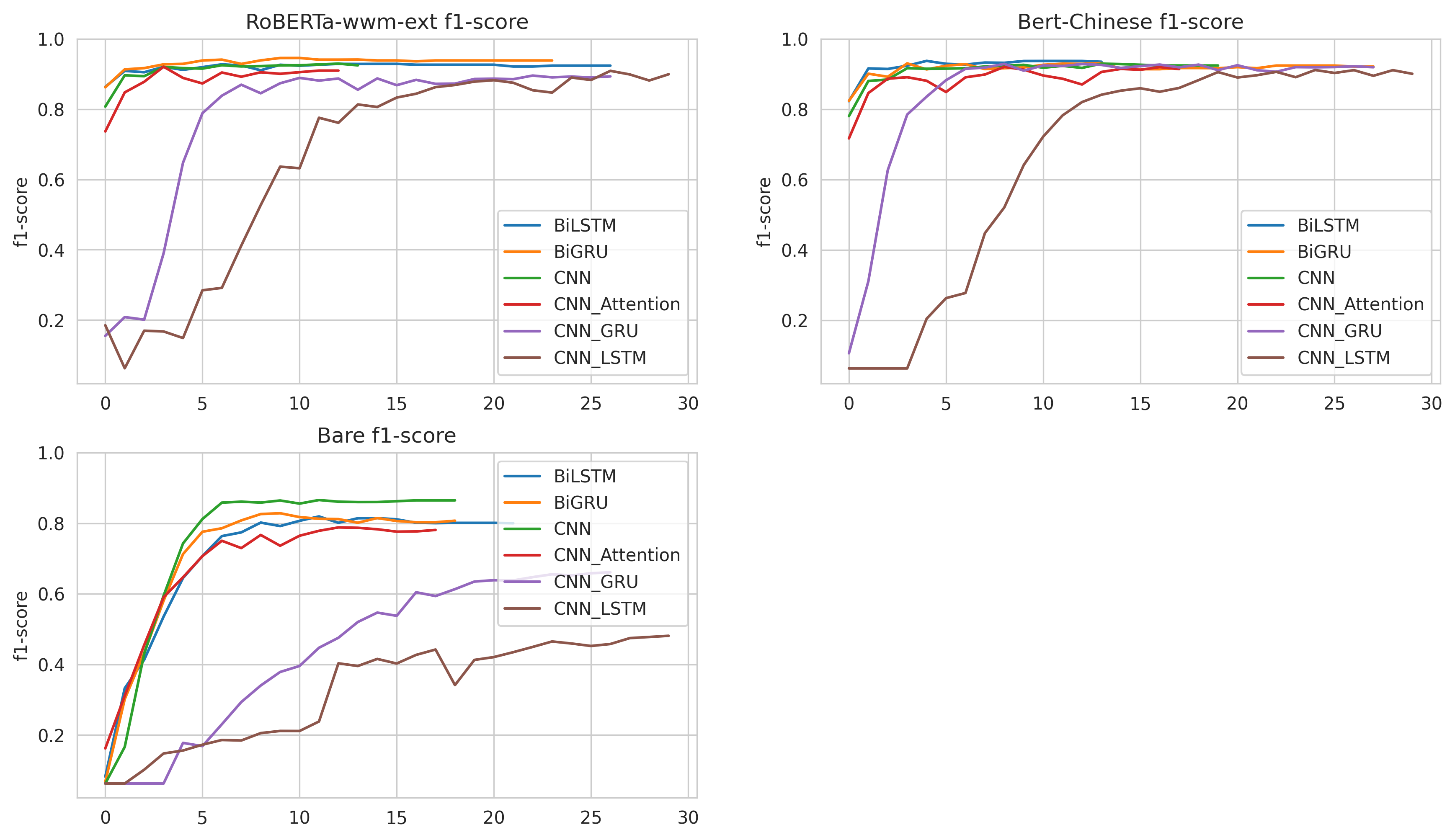Text Classification Model¶

Kashgari provides several models for text classification, All labeling models inherit from the ABCClassificationModel. You could easily switch from one model to another just by changing one line of code.

Available Models¶

Name info
BiLSTM_Model
BiGRU_Model
CNN_Model
CNN_LSTM_Model
CNN_GRU_Model
CNN_Attention_Model

Train basic classification model¶

Kashgari provides the basic intent-classification corpus for experiments. You could also use your corpus in any language for training.

from kashgari.corpus import SMP2018ECDTCorpus

# Or use your own corpus
train_x = [['Hello', 'world'], ['Hello', 'Kashgari']]
train_y = ['a', 'b']

valid_x, valid_y = train_x, train_y
test_x, test_x = train_x, train_y

Then train our first model. All models provided some APIs, so you could use any labeling model here.

import kashgari

import logging
logging.basicConfig(level='DEBUG')

model = BiLSTM_Model()
model.fit(train_x, train_y, valid_x, valid_y)

# Evaluate the model
model.evaluate(test_x, test_y)

# Model data will save to `saved_ner_model` folder
model.save('saved_classification_model')

# To continue training, compile the newly loaded model first
model.fit(train_x, train_y, valid_x, valid_y)

That’s all your need to do. Easy right.

Text classification with transfer learning¶

Kashgari provides varies Language model Embeddings for transfer learning. Here is the example for BERT Embedding.

import kashgari
from kashgari.embeddings import BertEmbedding

import logging
logging.basicConfig(level='DEBUG')

bert_embed = BertEmbedding('<PRE_TRAINED_BERT_MODEL_FOLDER>')
model = BiGRU_Model(bert_embed, sequence_length=100)
model.fit(train_x, train_y, valid_x, valid_y)

You could easily change model’s hyper-parameters. For example, we change the lstm unit in BiLSTM_Model from 128 to 32.

hyper = BiLSTM_Model.default_hyper_parameters()
print(hyper)
# {'layer_bi_lstm': {'units': 128, 'return_sequences': False}, 'layer_dense': {'activation': 'softmax'}}

hyper['layer_bi_lstm']['units'] = 32

model = BiLSTM_Model(hyper_parameters=hyper)

Use custom optimizer¶

from kashgari.corpus import SMP2018ECDTCorpus
# Remember to import kashgari before than RAdam

model = BiLSTM_Model()
# This step will build token dict, label dict and model structure
model.build_model(train_x, train_y, valid_x, valid_y)
# Compile model with custom optimizer, you can also customize loss and metrics.
model.compile_model(optimizer=optimizer)

# Train model
model.fit(train_x, train_y, valid_x, valid_y)

Use callbacks¶

Kashgari is based on keras so that you could use all of the tf.keras callbacks directly with Kashgari model. For example, here is how to visualize training with tensorboard.

from tensorflow.python import keras
from kashgari.callbacks import EvalCallBack

import logging
logging.basicConfig(level='DEBUG')

model = BiGRU_Model()

tf_board_callback = keras.callbacks.TensorBoard(log_dir='./logs', update_freq=1000)

# Build-in callback for print precision, recall and f1 at every epoch step
eval_callback = EvalCallBack(kash_model=model,
valid_x=valid_x,
valid_y=valid_y,
step=5)

model.fit(train_x,
train_y,
valid_x,
valid_y,
batch_size=100,
callbacks=[eval_callback, tf_board_callback])

Multi-Label Classification¶

Kashgari support multi-label classification, Here is how we build one.

Let’s assume we have a dataset like this.

x = [
['This','news','are' , 'very','well','organized'],
['What','extremely','usefull','tv','show'],
['The','tv','presenter','were','very','well','dress'],
['Multi-class', 'classification', 'means', 'a', 'classification', 'task', 'with', 'more', 'than', 'two', 'classes']
]

y = [
['A', 'B'],
['A',],
['B', 'C'],
[]
]

Now we need to init a Processor and Embedding for our model, then prepare model and fit.

import logging
from kashgari.embeddings import BertEmbedding

logging.basicConfig(level='DEBUG')

bert_embed = BertEmbedding('<PRE_TRAINED_BERT_MODEL_FOLDER>')

model = BiLSTM_Model(bert_embed, sequence_length=100, multi_label=True)
model.fit(x, y)

It is very easy and straightforward to build your own customized model, just inherit the ABCEmbedding and implement the default_hyper_parameters() function and build_model_arc() function.

from typing import Dict, Any

from tensorflow import keras

from kashgari.layers import L

import logging
logging.basicConfig(level='DEBUG')

class DoubleBLSTMModel(ABCClassificationModel):
"""Bidirectional LSTM Sequence Labeling Model"""

@classmethod
def default_hyper_parameters(cls) -> Dict[str, Dict[str, Any]]:
"""
Get hyper parameters of model
Returns:
hyper parameters dict
"""
return {
'layer_blstm1': {
'units': 128,
'return_sequences': True
},
'layer_blstm2': {
'units': 128,
'return_sequences': False
},
'layer_dropout': {
'rate': 0.4
},
'layer_time_distributed': {},
'layer_output': {

}
}

def build_model_arc(self):
"""
build model architectural
"""
output_dim = len(self.processor.label2idx)
config = self.hyper_parameters
embed_model = self.embedding.embed_model

layer_blstm1 = L.Bidirectional(L.LSTM(**config['layer_blstm1']),
name='layer_blstm1')
layer_blstm2 = L.Bidirectional(L.LSTM(**config['layer_blstm2']),
name='layer_blstm2')

layer_dropout = L.Dropout(**config['layer_dropout'],
name='layer_dropout')

layer_time_distributed = L.Dense(output_dim, **config['layer_output'])

# You need to use this actiovation layer as final activation
# to suppor multi-label classification
layer_activation = self._activation_layer()

# Define tensor flow
tensor = layer_blstm1(embed_model.output)
tensor = layer_blstm2(tensor)
tensor = layer_dropout(tensor)
tensor = layer_time_distributed(tensor)
output_tensor = layer_activation(tensor)

# Init model
self.tf_model = keras.Model(embed_model.inputs, output_tensor)

model = DoubleBLSTMModel()
model.fit(train_x, train_y, valid_x, valid_y)

Short Sentence Classification Performance¶

We have run the classification tests on SMP2018ECDTCorpus. Here is the full code: colab link

• SEQUENCE_LENGTH = 60

• EPOCHS = 30

• EARL_STOPPING_PATIENCE = 10

• REDUCE_RL_PATIENCE = 5

• BATCH_SIZE = 64

Embedding Model Best F1-Score Best F1 @ epochs
0 RoBERTa-wwm-ext BiLSTM_Model 92.89 15
1 RoBERTa-wwm-ext BiGRU_Model 94.57 10
2 RoBERTa-wwm-ext CNN_Model 92.95 12
3 RoBERTa-wwm-ext CNN_Attention_Model 92.07 3
4 RoBERTa-wwm-ext CNN_GRU_Model 89.56 22
5 RoBERTa-wwm-ext CNN_LSTM_Model 90.9 26
6 Bert-Chinese BiLSTM_Model 93.74 4
7 Bert-Chinese BiGRU_Model 93.12 13
8 Bert-Chinese CNN_Model 92.95 13
9 Bert-Chinese CNN_Attention_Model 92.04 8
10 Bert-Chinese CNN_GRU_Model 92.88 8
11 Bert-Chinese CNN_LSTM_Model 91.15 24
12 Bare BiLSTM_Model 81.96 11
13 Bare BiGRU_Model 82.86 9
14 Bare CNN_Model 86.61 11
15 Bare CNN_Attention_Model 78.84 12
16 Bare CNN_GRU_Model 66.14 26
17 Bare CNN_LSTM_Model 48.13 29